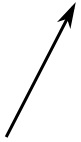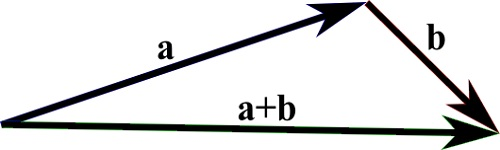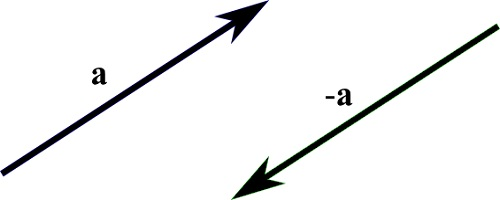# Vectors

Vectors are 1-dimentional Arrays

Vectors have a Magnitude and a Direction

Vectors typically describes Motion or Force

## Vector Notation

Vectors can be written in many ways. The most common are:

v =
 1 2 3

or:

v =
 1 2 3

## Vectors in GeometryThe image to the left is a Vector. The Length shows the Magnitude. The Arrow shows the Direction.

## Motion

Vectors are the building blocks of Motion

In geometry, a vector can describe a movement from one point to another.

The vector [3, 2] says go 3 right and 2 up.

The sum of two vectors (a+b) is found by moving the vector b until the tail meets the head of vector a. (This does not change vector b).

Then, the line from the tail of a to the head of b is the vector a+b:## Vector Subtraction

Vector -a is the opposite of +a.

This means that vector a and vector -a has the same magnitude in opposite directions:## Scalar Operations

Vectors can be modified by adding, subtracting, or multiplying a scalar (number) from all the vector values:

a = [1 1 1]

a + 1 = [2 2 2]

[1 2 3] + 1 = [2 3 4]

Vector multiplications has much of the same properties as normal multiplication:

[2 2 2] * 3 = [6 6 6]

[6 6 6] / 3 = [2 2 2]

## Force

Force is a Vector.

Force is a vector with a Magnitude and a Direction.

## Velocity

Velocity is a Vector.

Velocity is a vector with a Magnitude and a Direction.

W3Schools is optimized for learning and training. Examples might be simplified to improve reading and learning. Tutorials, references, and examples are constantly reviewed to avoid errors, but we cannot warrant full correctness of all content. While using W3Schools, you agree to have read and accepted our terms of use, cookie and privacy policy.